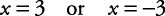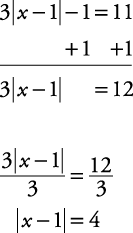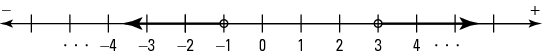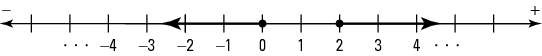## Solving Equations Containing Absolute Value

To solve an equation containing absolute value, isolate the absolute value on one side of the equation. Then set its contents equal to both the positive and negative value of the number on the other side of the equation and solve both equations.

##### Example 1

Solve | x | + 2 = 5.

Isolate the absolute value.Set the contents of the absolute value portion equal to +3 and –3.##### Example 2

Solve 3| x – 1| – 1 = 11.

Isolate the absolute value.Set the contents of the absolute value portion equal to +4 and –4.

Solving for x,#### Solving inequalities containing absolute value and graphing

To solve an inequality containing absolute value, begin with the same steps as for solving equations with absolute value. When creating the comparisons to both the + and – of the other side of the inequality, reverse the direction of the inequality when comparing with the negative.

##### Example 3

Solve and graph the answer: | x – 1| > 2.

Notice that the absolute value expression is already isolated.

| x – 1| > 2

Compare the contents of the absolute value portion to both 2 and –2. Be sure to reverse the direction of the inequality when comparing it with –2.

Solve for x.Graph the answer (see Figure 1).

Figure 1. The graphic solution to | x – 1| > 2.##### Example 4

Solve and graph the answer: 3| x| – 2 ≤ 1.

Isolate the absolute value.Compare the contents of the absolute value portion to both 1 and –1. Be sure to reverse the direction of the inequality when comparing it with –1.Graph the answer (see Figure 2).

Figure 2. Graphing the solution to 3| x| – 2 ≤ 1.##### Example 5

Solve and graph the answer: 2|1 – x| + 1 ≥ 3.

Isolate the absolute value.Compare the contents of the absolute value portion to both 1 and –1. Be sure to reverse the direction of the inequality when comparing it with –1.

Solve for x.(Remember to switch the direction of the inequality when dividing by a negative)Graph the answer (see Figure 3).

Figure 3. Graphing the solution 2|1 – x| + 1 ≥ 3.